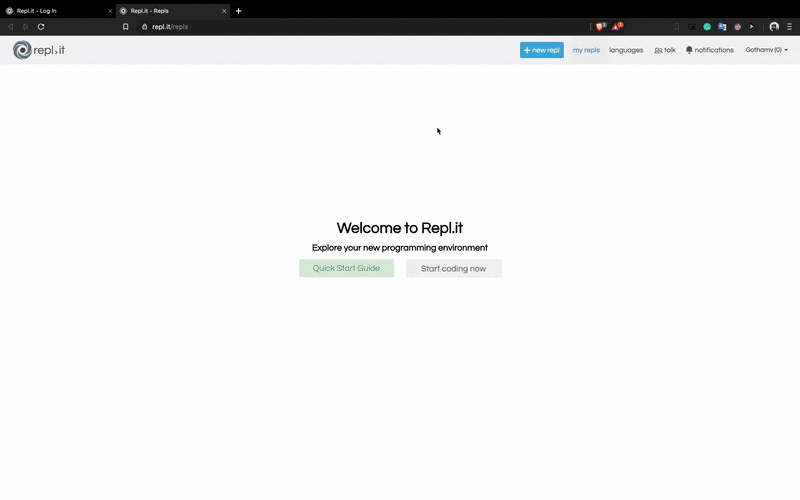# Part I: Setting up the coding environmentFollow the GIF if you have any confusions.

# 1. Let’s print stuff using Python :

`print('Hello World!')print("Hello World!")`

# 3. Variables in Python

## Let’s assign a number to a variable.

`myVariable = 13myVariable2 = 12.6969`
`type(myVariable)type(myVariable2)# To view this in the console pass the above statement inside the print function like this :print(type(myVariable))print(type(myVariable2))`

## Let’s assign a character/string to a variable.

`char1 = 'a'string1 = "Musk Cult"`

# 4. Arithmetic Operations in Python

`myVariable1 = 6myVariable2 = 3`

## Addition + :

`myVariable + myVariable2# To see the result on the console :print(myVariable + myVariable2)`

## Subtraction - :

`print(myVariable - myVariable2)`

## Multiplication * :

`print(myVariable * myVariable2)`

## Division / :

`print(myVariable / myVariable2)`

# 5. Printing variables

`result = myVariable + myVariable2print(result)`
`print("The result of addition is :",result)`
`print("%s is the value of myVar!" % myVar)`
`print("The result of addition of %s and %s is : %s" % (myVar,myVar2,result))`

# 6. Getting input from the user.

`myVar = input("Enter the First Number : ")myVar2 = input("Enter the Second Number : ")`
`myVar = int(input("Enter the First Number : "))myVar2 = int(input("Enter the Second Number : "))`

# Peace Out ✌️

I write about Tech. Algorithms. Programming. And that kinda thing you know?

## More from Gowtham Venkatesan

I write about Tech. Algorithms. Programming. And that kinda thing you know?